Technical Article

# How to Monitor Current with an Op-Amp, a BJT, and Three Resistors

February 19, 2018 by Robert Keim

## This article, part of AAC’s Analog Circuit Collection, explains the functionality of a clever circuit that accurately measures supply current.

This article, part of AAC’s Analog Circuit Collection, explains the functionality of a clever circuit that accurately measures supply current.

First of all, I have to admit that the title is slightly misleading. The circuit presented in this article does indeed require only an op-amp, a transistor, and three resistors. It is not, however, a self-contained current monitor in the sense that it measures current and initiates actions based on the measurements. So maybe “current measurer” would be more accurate than “current monitor,” but even “current measurer” doesn’t quite capture it because the circuit doesn’t record the current values or convert them into a visual indication.

In the end, I suppose the circuit is little more than a “current-to-voltage converter,” but keep in mind that it converts current to voltage in a way that is compatible with supply-current-monitoring applications. So maybe we should call it a “current-to-voltage converter for power-supply-current-delivery-monitoring applications” (abbreviated CTVCFPSCDMA). Perfect.

### Why?

There are various situations in which you might want to measure the current consumed by your design. Maybe you want to dynamically adjust the functionality of one subsystem based on the current consumption of another subsystem. Maybe you’re trying to estimate battery life, or to establish the smallest-possible regulator IC that can provide adequate output current. You could even use recorded current-consumption measurements as a minimally invasive way of tracking a microcontroller’s transitions between higher- and lower-power states.

### How?

As discussed in the opening paragraphs, this circuit converts current to voltage. This might fulfill your current-monitoring requirements if all you need to do is manually observe current-consumption behavior using a multimeter or an oscilloscope. I suppose you could even record and analyze your current-consumption measurements using a data-acquisition device and some appropriate software.

If you need a circuit that is more autonomous in its ability to record and/or respond to current consumption behavior, you’ll probably want to digitize the measurements using a microcontroller. If only basic functionality is required and you have no other need for a processor, you could use a comparator or an analog window detector.

### The Circuit

The CTVC... presented in this article is based on a circuit found in an application note entitled “Op Amp Circuit Collection,” published (back in 2002) by National Semiconductor. My version looks like this: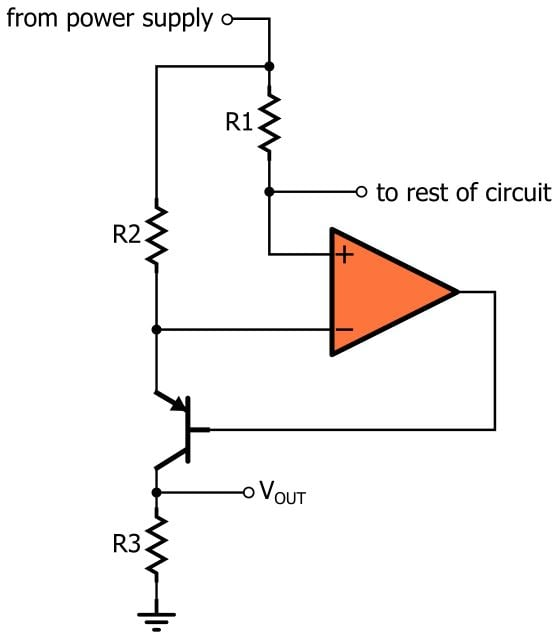And here is my LTspice implementation: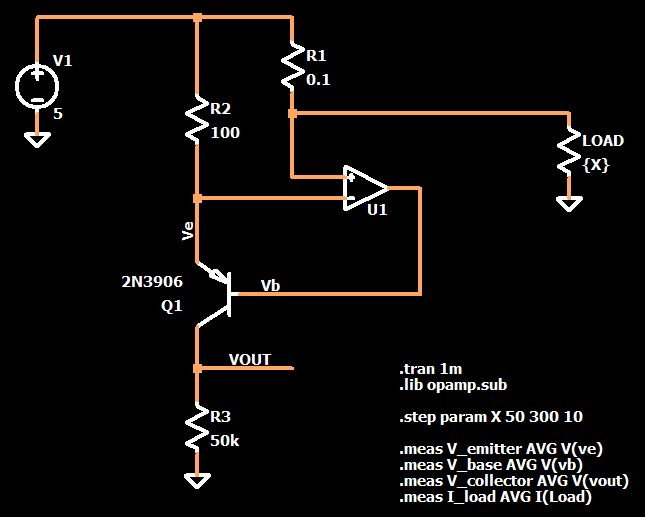This may look a bit confusing at first glance, but the operation really is rather straightforward. Let’s walk though it:

• The current flows from the power supply, through R1, to the load. R1 functions as a typical current-sensor resistor, and like other current-sense resistors it has a very low resistance so as to reduce power dissipation and minimize its effect on the measurements and on the load circuit.
• The voltage applied to the op-amp’s non-inverting input terminal is equal to the supply voltage minus (supply current × R1).
• Don’t let the PNP transistor distract you from the fact that the op-amp does indeed have a negative feedback loop. The presence of negative feedback means that we can apply the virtual short approximation, i.e., we can assume that the voltage at the inverting input terminal is equal to the supply voltage minus (supply current × R1).
• Since the upper terminal of both R1 and R2 is tied to the supply voltage, the virtual-short assumption tells us that an equal voltage appears across both of these resistors, and consequently the current through R2 is equal to the current through R1 divided by the ratio of R2 to R1. In the LTspice circuit shown above, R2 is 1000 times larger than R1, which means that the current through R2 will be 1000 times smaller than the current through R1.
• The BJT’s base current is very small, so we can say that the current through R3 is more or less equal to the current through R2. Thus, we use R3 to create a voltage that is directly proportional to the current through R2, which in turn is directly proportional to the current through R1.

Here’s a diagram that should help to clarify and reinforce this explanation: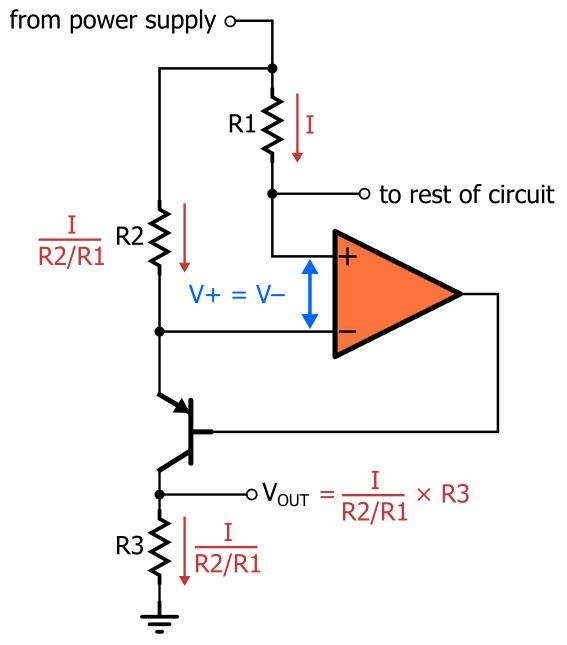As you can see, the final equation for VOUT is

$$V_{OUT}=\frac{I_{LOAD}}{R2/R1}\times R3 = \frac{R1\cdot R3}{R2}\times I_{LOAD}$$

### What Exactly Is That PNP Doing...?

You can think of the transistor in two ways: as an adjustable valve that allows the op-amp to increase or decrease the current flowing through R2 and R3, or as a variable voltage-dropping device that the op-amp can use to establish the correct voltage at the VOUT node. In both cases the end result is the same: the transistor is the means by which the op-amp can force the voltage at the inverting input terminal to equal the voltage at the non-inverting input terminal.

The transistor really is the most interesting part of this circuit. We often use BJTs in “on or off” applications, and it’s important to recognize that the situation in this circuit is completely different. The op-amp (with the help of negative feedback, of course) is actually making small, precise adjustments to the PNP’s emitter-to-base voltage (VEB). The following plot shows VEB for a range of load currents (corresponding to load resistances from 50 Ω to 300 Ω).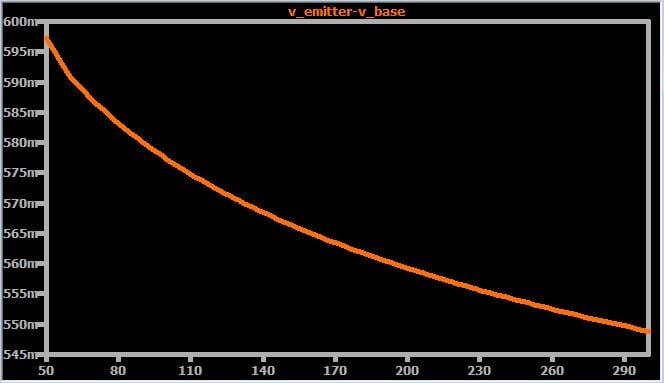##### For information on how to create a plot with a stepped parameter on the horizontal axis, see this page.

Notice how all of these voltages are near the typical turn-on threshold (~0.6 V) for a silicon pn junction. What this tells you is that the op-amp is very carefully negotiating the BJT’s threshold region in order to produce the required—and relatively large—changes in the emitter-to-collector voltage drop. The entire range of VEB values is only ~50 mV, and compare this ~50 mV variation to the ~4 V variation in emitter-to-collector voltage: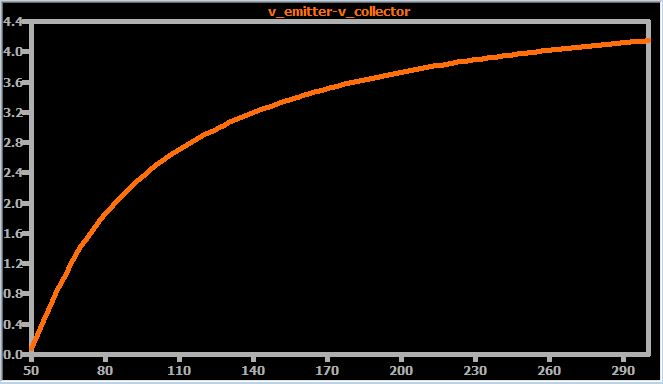### Performance

Real implementations of this circuit will, of course, have error sources that cause the load current vs. output voltage relationship to deviate from the ideal formula given above. Even the LTspice circuit isn’t quite perfect because of the realistic behavior incorporated into the BJT model (and perhaps the op-amp model as well). However, if you have high-precision resistors and a good op-amp, I think that this circuit can be quite accurate. The following plot conveys the simulated error over the same load-resistance range (keep in mind that “V_collector” is the same as VOUT).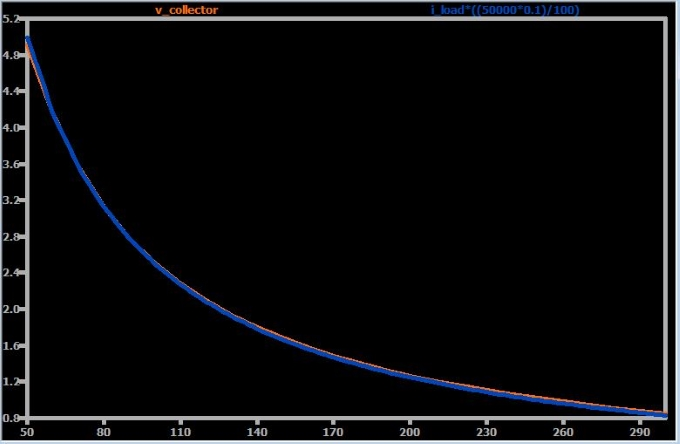The two traces almost perfectly overlap, indicating good accuracy. Notice how the orange trace is noticeably lower than the blue trace at the smallest resistance value; this occurs because a load resistance of 50 Ω corresponds to an output voltage of 5 V, but VOUT cannot be exactly 5 V because at least a little bit of voltage must be dropped across R2 and across the emitter-collector junction.

### Conclusion

We’ve covered an interesting and effective circuit that accurately converts supply current into a voltage that can be measured, digitized, or used as an input to a comparator. If you would like to continue exploring this handy circuit, feel free to save yourself a bit of work by downloading my LTspice schematic (just click on the orange button).

Op-amp_current_monitor.zip

• Share• S
ShirEl February 21, 2018

NIce !!!

Like.
• P
patlaw February 27, 2018

This explanation is excellent. It is very helpful in understanding these circuits. However, I still don’t fully understand the design philosophy behind this circuit with regard to the BJT. In particular, when analyzing a circuit, I have a problem identifying what the “absolutes” are. For example, the op amp wants to maintain the same voltage at the inverting and non-inverting inputs. The EB junction of the BJT is somewhat fixed at approximately 0.6v. The section, “What Exactly Is That PNP Doing…” was exactly what I wanted to see in that part of the article, but I’m still missing some nuance in the use of the BJT.

Like.
•RK37 April 18, 2018
The fundamental concept here is that the EB junction is not fixed at 0.6 V. This widespread simplification can be highly problematic because it is so inconsistent with reality. A pn junction has an exponential current-voltage relationship. The transition from "off state" to "on state" seems abrupt by the standards of human perception, but an op-amp equipped with negative feedback can easily navigate within the transition region so as to produce the required base-emitter voltage (which in turn influences the collector-emitter voltage). I think these sentences from the article capture it pretty well; maybe not, or maybe they didn't catch your eye as you were reading through: "Notice how all of these voltages are near the typical turn-on threshold (~0.6 V) for a silicon pn junction. What this tells you is that the op-amp is very carefully negotiating the BJT’s threshold region in order to produce the required—and relatively large—changes in the emitter-to-collector voltage drop."
Like.
•Martin Green April 24, 2018
The BJT is simply controlling the current through R2/R3 such that the voltage across R2 is the same as across R1. Assuming a perfect BJT with infinite hfe, the current through R3 is identical to that through R2, which allows us to read the R2/R3 current as a voltage across R3. There is nothing magical about the BE voltage drop in this circuit, and better versions of it I have linked to in another reply here dispense with the BJT and use a JFET or MOSFET instead since the base current required to control the R2/R3 current will introduce an error on the output voltage. The BJT simply gives the op-amp a way to control the current flowing though R2 and thus also through R3.
Like.
•Andre Gulbis March 06, 2018

I wonder how accurate you could get this?  I guess with good components and maybe some calibration in digital it could be very good?

Like.
• H
Harold541 April 13, 2018
Yep, program the curve into a micro's A/D and with an LCD you could have a measurement.,......
Like.
•Martin Green April 24, 2018
There is no "curve" to program. The voltage on R3 is linearly proportional to the current through R1. The errors come from three sources... most important is the base current of Q1. Any current from the op-amp output which flows into the base of Q1 will also flow through R3 and add a little excess voltage to the output. The solution to this is to choose the highest hfe transistor you can find. Even better is to replace it with a darlington pair for hfe measured in the tens-of-thousands instead of hundreds. For the best results get rid of the BJT entirely and use a FET instead since FETs have effectively zero gate current. The other primary error source doesn't affect linearity, but if you want accurate readout of R1 current, the ratios of R1/R2/R3 must be precise so the current through R3 produces the correct voltage at the output. Replacing R2 with a 10-turn pot allows precise calibration if you have an accurate ammeter on the output to compare to. Finally, the op-amp selection is important too. Generally, the higher the open-loop gain the better, but even a super high gain won't overcome errors if the device inputs aren't well balanced. This basic design has been around for almost 50 years, and a FET version of it appears in Texas Instruments app note 32 from 1970 using an LM301A op-amp and 2N3684 FET. http://www.ti.com/lit/an/snoa620/snoa620.pdf Another TI app note, #31, (dated 2002 despite its lower number) implements it with an LM107 and a MOSFET. https://www.ti.com/ww/en/bobpease/assets/AN-31.pdf
Like.
• R
ronsoy2 April 24, 2018

Clever, but limited in the real world. This exact problem came up with a 5 output power supply with the highest voltage supply varying from zero to 350 volts. Instantly, you see that multiple problems arise! Note that the OPamp must follow the output voltage! This means somewhere to get supply voltage for it, both above and below the output voltage. If the main supply is always above a certain level, another current source and zener could be used, but this fails if the main monitored supply output includes zero. In the end, an isolated DC-DC converter was used.
The linearity of the circuit is directly affected by the OPamp. For large currents today’s super low offset OPamps can easily do 1% linearity, but if you want to measure 1/10% at milliamp output current levels you will have big problems even with the super OPamps with microvolt offsets. I was unable to get the bottom digit of a 4 1/2 digit panel meter to be accurate using the circuit. The lesson here is DON’T believe what LTspice says until you actually build a real prototype and are faced with the real world complications of the design.

Like.
• F
farmerkeith April 30, 2018

Unless you use an additional power supply, the Op amp has to be capable of a) operating at the full voltage of the current shunt, relative to ground; and b) providing its differential gain with its inputs at the high rail voltage; and c) generating its output voltage within Vbe of the rail.
So for example if you want to use this circuit to measure the current in a typical solar panel with 60 cells in series, the rail voltage can be nearly 40 Volts, so you need a rail-to-rail op amp with a 40 volt rating. These do exist.
The rail-to-rail output requirement would be removed by using a

Like.
• F
farmerkeith April 30, 2018
(continuation, I must have hit a wrong button) using an enhancement mode MOSFET instead of a BJT as suggested in one of the other comments. This should also improve tracking accuracy. I think the SPICE simulation would better show the current measuring capability by using a voltage for the load on the right hand side and a current generator on the left hand side of the current shunt resistor.
Like.
• P
pekka123 July 11, 2018

Robert Keim

I liked your article How to Monitor Current with an Op-Amp, a BJT, and Three Resistors
But have you actually built the circuit?
What is the opamp?

You may know that not all opamps work with power supply range like LM324 and and LM321.

Pekka Ritamaki Finland

Like.
•RK37 July 12, 2018
No, I didn't build the circuit. The Analog Circuit Collection articles are not intended to explain or evaluate a specific implementation. They present a general topology and some generic simulations; the details of a real-world implementation will vary according to the conditions and requirements of each application.
Like.
•377Ohms November 10, 2018

Does this circuit work with the op-amp powered from the single supply? If not, how to make it work single-supply?

Like.
• J
jacobw56 January 23, 2019

Has anyone actually built this circuit successfully? I have put it together with the BJT specified here, as well as a handful of others, and several different precision rail-to-rail op amps and I never get anything resembling the simulation.  Typically I see V_out rise for a bit then collapse to ground, where it stays indefinitely.  Talk about reality departing from the ideal….

Like.